Poisson’s & Laplace’s Equations

# Poisson’s & Laplace’s Equations - Notes | Study Electromagnetic Fields Theory (EMFT) - Electrical Engineering (EE)

 1 Crore+ students have signed up on EduRev. Have you?

We will spend some time in looking at the mathematical foundations of electrostatics. For a charge distribution defined by a charge density ρ, the electric field in the region is given by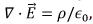which gives, for the potential φ, the equation which is known as the Poisson’s equation,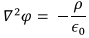In particular, in a region of space where there are no sources, we have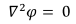Which is called the Laplace’s equation. In addition, under static conditions, the equation is valid everywhere.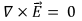Solutions of Laplace’s equation are known as Harmonic functions. The expressions for the Laplacian operator in Cartesian, spherical and cylindrical coordinates are given by the following expressions :

Cartesian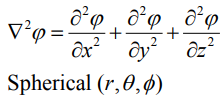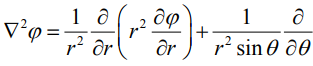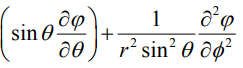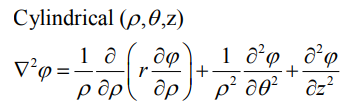(It may be noted that we have used the symbol φ for the potential and a closely similar symbol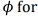the azimuthal angle of the spherical coordinate system, which should not cause confusion).

The formal solution of Poisson’s equation has been known to us from our derivation of the form of potential using Coulomb’s law. It is easy to check that the expression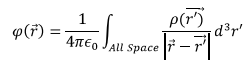Where we have used the primed variable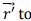indicate the integrated variable and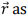the position coordinate of the point where the potential is calculated. We will operate both sides of the above equation with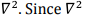acts on the variable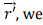can take it inside the integral on the right and make it operate on the function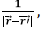and get,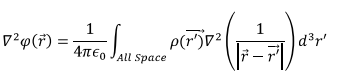Recall that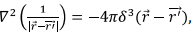so that the integral on the right is easily computed using the property of the delta function to be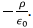Uniqueness Theorem :

There may be many solutions to Poisson’s and Laplace’s equations. However, the solutions that interest us in Physics are those which satisfy the given boundary conditions. Other than the assumed existence of the solutions subject to given boundary conditions, one theorem that comes to our help is what is known as the Uniqueness Theorem. The theorem basically states that corresponding to various possible solutions of Laplace’s or Poission’s equation, the solution that satisfies the given boundary condition is unique, i.e., no two different solutions can satisfy the given equation with specified boundary conditions. The great advantage of the theorem lies in the fact that if we obtain a solution by some technique or even by intuition, we need not look any further, the solution that we have at hand is the only possible solution.

What are these boundary conditions? Consider, for instance, a situation in which we are required to find the solution of either of these equations in a region of volume V bounded by a surface S. Within this region, we have, for instance, a set of conductors with surfaces S1, S2, … on which we know the values of the potential. In addition, we are given the potential function on the surface S itself. This is an example of boundary condition, known as the Dirichlet boundary condition.

It is also possible that instead of specifying the values of potential on the conductors, we are given the electric fields on the surface of the conductors, which as we know, are directed normal to the surface of the conductors. This would be yet another example of boundary conditions. Boundary conditions where the normal derivatives of the function to be derived are specified on some surface or surfaces are known as the Neumann boundary condition.

In addition to these two types of boundary conditions, there are other possible boundary conditions. For instance in Cauchy boundary condition, the value of both the potential and its normal derivative are specified. There could be mixed boundary conditions in which different types of boundary conditions may be specified on different parts of the boundary.

Uniqueness theorem applies to those cases where there is only one type of boundary condition, viz. either Dirichlet or Neumann boundary condition.

To prove the uniqueness theorem, let us assume that contrary to the assertion made in the theorem, there exist two solutions φ1 and φ2 of either Poisson’s or Laplace’s equation which satisfy the same set of boundary conditions on surfaces S1, S2, … and the boundary S. The conditions, as stated above, may be either of Dirichlet type or Neumann type :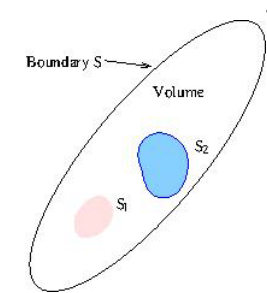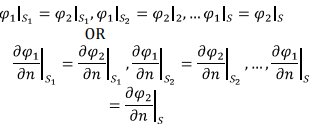Let us define a new function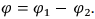In view of the fact that φ1 and φ2 satisfy the same boundary conditions, the boundary condition satisfied by φ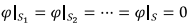(Dirichlet) OR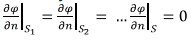(Neumann).

Further, whether φ1 and φsatisfy Poisson’s or Laplace’s equation, their difference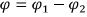satisfies Laplace’s equation.

In order to prove the Uniqueness theorem we will use Green’s First Identity, derived in Module 1, which states that for two arbitrary scalar fields φ and ψ, the following identity holds,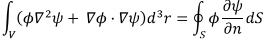where S is the boundary defining the volume V. We choose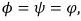to get,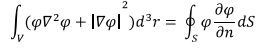Since on the surface , either the Dirichlet or the Neumann boundary condition is valid, the right hand side of the above is zero everywhere. (Note that the surface consists of S + S1 + S2 + ...., with the direction of normal being outward on S and inward on the conductor surfaces enclosed).

Since φ satisfies Laplace’s equation, we are then left with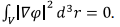The integrand, being a square of a field is positive everywhere in the volume and its integral can be zero only if the integral itself is identically zero. Thus we have,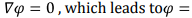constant φ = 0 on the surface and therefore, it is zero everywhere in the volume giving,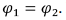If, on the other hand, Neumann boundary condition is satisfied, we must have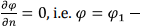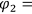constant everywhere. Since the constant can be chosen arbitrarily, we take the constant to be zero and get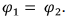Example 1 : Parallel Plate Capacitor :

Consider a parallel plate capacitor with a plate separation of d between the plates. The lower plate is grounded while the upper plate is maintained at a constant potential φ0. Between the two plates there are no sources and hence Laplace’s equation is valid in this region. Since the plates are assumed infinite in the x, y directions, the only variation is with respect to the z direction and we have,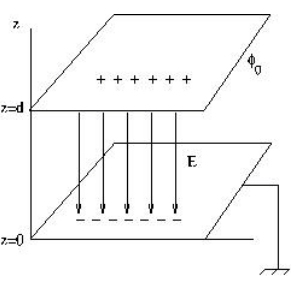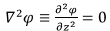The solution of this equation is straightforward, and we get,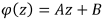. where A and B are constants. Substituting the boundary conditions at z=0 and at z=d,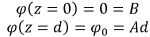which gives,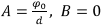Substituting these, we get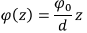The electric field in the region is given by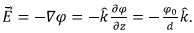This shows that the electric field between the plates is constant and is directed from the upper plate toward the lower plate. The upper plate gets positively charged and the lower plate is negatively charged. We can find out the charge densities on the plates by taking the normal component of the electric field. For instance on the upper plate, the direction of normal being in the negative z direction,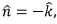we get,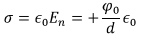Likewise, the normal to the lower plate being in the positive z direction, the charge density on that plate is equal and opposite. If we multiply the charge density by the area of the plate A, we get (area is taken to be large so that the edge effects are neglected),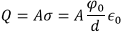to be the charge on the upper plate. The lower plate has equal but opposite charge. Dividing the amount of charge on the positive plate by the potential difference φ0 between the plates, we get the capacitance of the parallel plate capacitor to be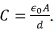Example 2 : Coaxial Cable :

Consider a coaxial cable of inner radius a and outer radius b. The outer conductor is grounded while the inner conductor is maintained at a constant potential φ0 Taking the z axis of the cylindrical coordinate system along the axis of the cylinders, we can write down the Laplace’s equation in the space between the cylinders as follows :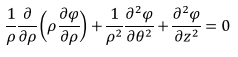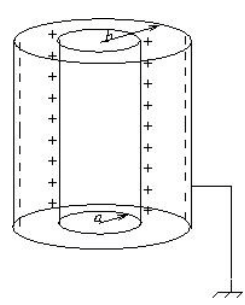Here p is the radial distance from the axis and θ is the polar angle. We assume the cylinders to be of infinite extend in the z direction. This implies that there is azimuthal symmetry and no variation with respect to z as well. The solution can only depend on the radial distance p. Giving.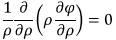The solution of the above equation is easy to obtain, and we get,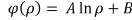where A and B are two constants which must be determined from the boundary conditions.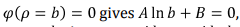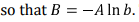The boundary condition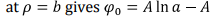In b,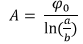Substituting these in the expression for the potential, we get the potential in the region between the cylinders to be given by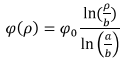The electric field in the region is given by the negative gradient of the potential which is simply the derivative with respect to p,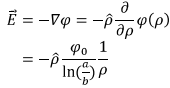Since In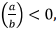the direction of the electric field is outward from the inner conductor, which gets positively charged. The charge density of the inner conductor is given by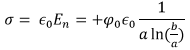where we have taken care of the minus sign by inverting the argument of log. Note that the expression for the charge density on the outer plate will not be identical because of the fact that the radii of the two cylinders are different. The total charge per unit length on the inner conductor is given by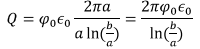The magnitude of the charge per unit length on the outer conductor can be seen to be the same. The capacitance per unit length is thus given by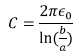Example 3 : Spherical Capacitor:

Consider a spherical capacitor with the inner conductor having a radius a and the outer conductor a radius b. The outer conductor is grounded and the inner conductor is maintained at a constant potential φ0 . Because of spherical symmetry, the potential can only depend on the radial distance r. The radial part of Laplace’s equation, which is valid in the space between the conductors is given by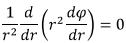This is a differential equation in a single variable, and the solution can be easily obtained as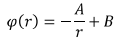where A and B are constants.

Inserting the boundary conditions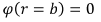gives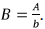The other boundary condition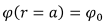gives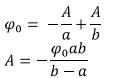Substituting these into the solution, we have,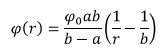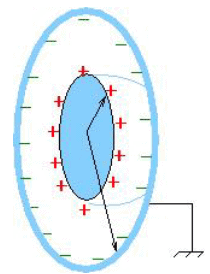As before, we find the charge density on the spheres by taking the normal component of the electric field. The electric field is given by,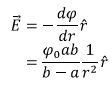The charge density on the inner plate is given by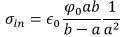The total charge on the inner sphere is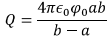It can be checked that the outer sphere has equal and opposite negative charge. The capacitance is given by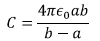The capacitance of a single spherical conductor is obtained by taking the outer sphere to infinity, i.e.,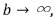which gives the capacitance for a single conductor to be

This result is not surprising because for a single conductor containing charge Q, the potential is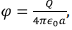so that the capacitance, which is given by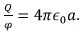Tutorial Assignment

1.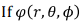satisfies Laplace’s equation inside a sphere, show that the average of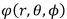over the surface is equal to the value of the potential at the origin.

2. Consider two concentric spherical shells of radii R1 and R2 . A charge Q1 resides on the inner sphere and Q2 on the outer sphere. The potential is set to be zero at infinite distance. Find the potential at the centre of the sphere.

Solutions to tutorial assignment

1. The average of the potential over the surface is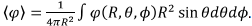Since the angles are averaged out, the right hand side can only depend on R. Thus we have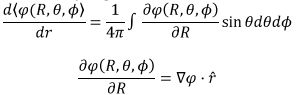Since the outward normal on the sphere is along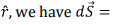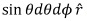and we can write,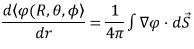The right hand side can be converted to a volume integral using the divergence theorem,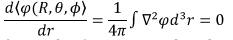sincesatisfies Laplace’s equation. Thus the average in independent of the radius of the sphere and we can continuously decrease the size of the sphere so that it reduces to a point at the origin.

2. The potential on the surface of the inner sphere can be easily shown to be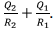This must also be the potential at the origin by uniqueness theorem as derived in Problem 3.

Self Assessment Quiz

1. If φ is a solution of Laplace’s equation, show that one or more derivatives of φ with respect to rectangular coordinates also satisfy Laplace’s equation.

2. Using the property of solutions to Laplace’s equation show that there cannot be an electrostatic field inside a hollow conductor unless there is a charge in that region.

3. A conductor has a hollow scooped out inside. The conductor carries a charge and the potential of the conductor is φ0 with respect to its zero at infinity. Show that the potential inside the hollow is also φ0 .

4. Consider two point conductors separated by a distance of 10m. One of the conductors is maintained at a potential of 100 V while the other maintained at 200 V. Determine the potential function and the corresponding electric field..

Solutions to Self Assessment Quiz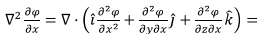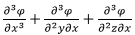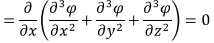2. The inner surface of the conductor which encloses the hollow is an equipotential. Let the potential be φ0 . If there are no charges in the hollow, the potential there must satisfy Laplace’s equation. We know that solutions to Laplace’s equations have no local minima or maxima. Thus the potential inside the hollow must be the same as that on its boundary, i.e., the potential has a constant value φ0 . Thus the electric field inside the hollow is zero.

3. Conductor is an equipotential. Thus the inner surface of the hollow is also at a potential φ0 . The potential inside the hollow must have the same value of the potential because (i) being a constant, it satisfies Laplace’s equation and (ii) it satisfies Dirichlet boundary condition on the surface. By uniqueness theorem, this is the only possible solution.

4. Let the first conductor be located at the origin and the second at x=10. On the entire x axis other than at these two points, Laplace’s equation is applicable, which has the solution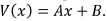Inserting boundary conditions we have,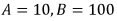so that the potential is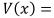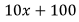Volts. The electric field is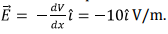The document Poisson’s & Laplace’s Equations - Notes | Study Electromagnetic Fields Theory (EMFT) - Electrical Engineering (EE) is a part of the Electrical Engineering (EE) Course Electromagnetic Fields Theory (EMFT).
All you need of Electrical Engineering (EE) at this link: Electrical Engineering (EE)

## Electromagnetic Fields Theory (EMFT)

11 videos|46 docs|62 tests
 Use Code STAYHOME200 and get INR 200 additional OFF

## Electromagnetic Fields Theory (EMFT)

11 videos|46 docs|62 tests

Track your progress, build streaks, highlight & save important lessons and more!

,

,

,

,

,

,

,

,

,

,

,

,

,

,

,

,

,

,

,

,

,

;